Anzeige

# Design of sliding mode controller for chaotic Josephson-junction

IJECEIAES
21. Mar 2023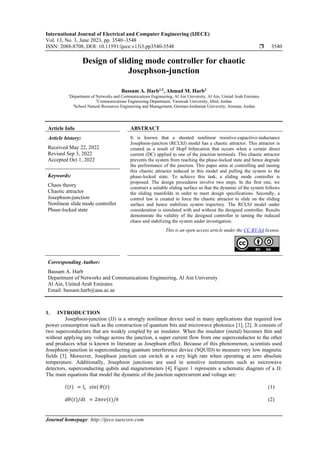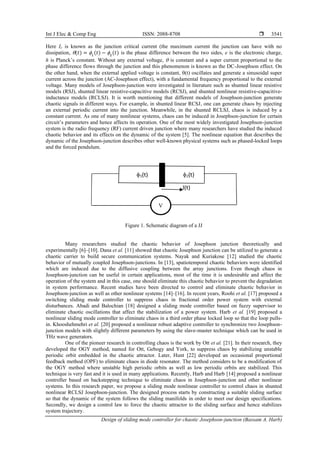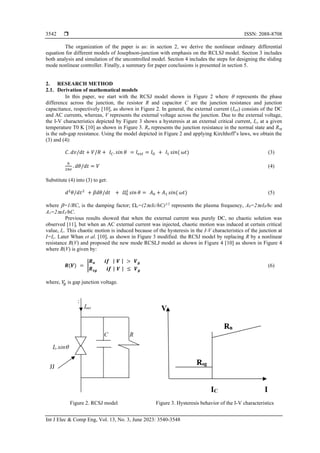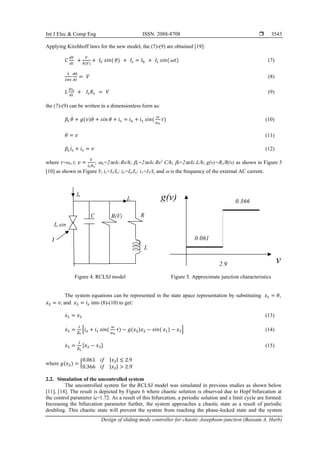Anzeige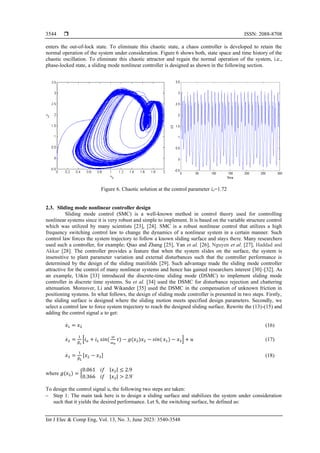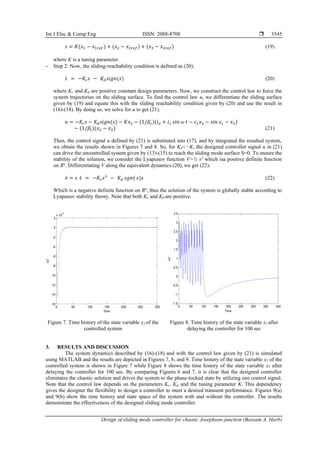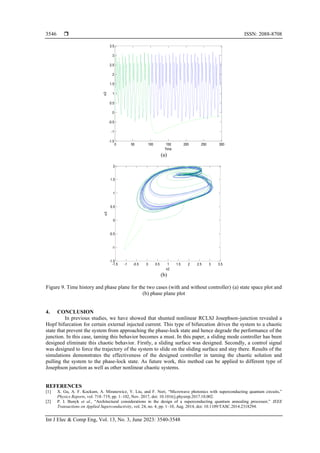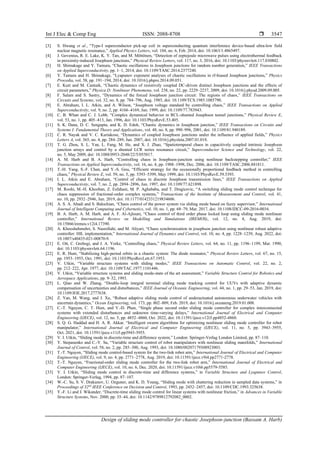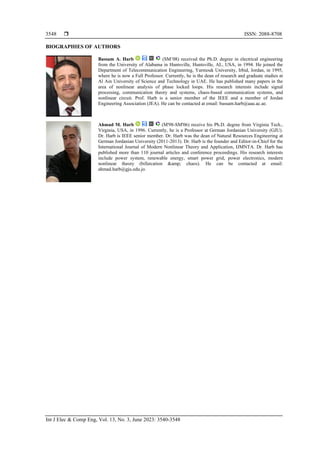Nächste SlideShare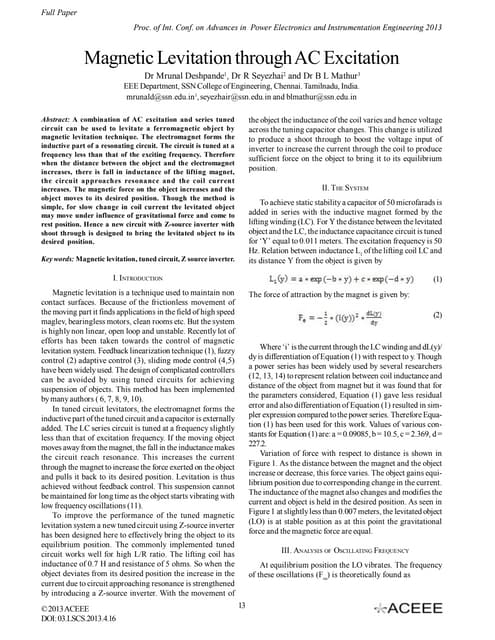Magnetic Levitation through AC Excitation
1 von 9
Anzeige

### Design of sliding mode controller for chaotic Josephson-junction

1. International Journal of Electrical and Computer Engineering (IJECE) Vol. 13, No. 3, June 2023, pp. 3540~3548 ISSN: 2088-8708, DOI: 10.11591/ijece.v13i3.pp3540-3548  3540 Journal homepage: http://ijece.iaescore.com Design of sliding mode controller for chaotic Josephson-junction Bassam A. Harb1,2 , Ahmad M. Harb3 1 Department of Networks and Communications Engineering, Al Ain University, Al Ain, United Arab Emirates 2 Communications Engineering Department, Yarmouk University, Irbid, Jordan 3 School Natural Resources Engineering and Management, German-Jordanian University, Amman, Jordan Article Info ABSTRACT Article history: Received May 22, 2022 Revised Sep 3, 2022 Accepted Oct 1, 2022 It is known that a shunted nonlinear resistive-capacitive-inductance Josephson-junction (RCLSJ) model has a chaotic attractor. This attractor is created as a result of Hopf bifurcation that occurs when a certain direct current (DC) applied to one of the junction terminals. This chaotic attractor prevents the system from reaching the phase-locked state and hence degrade the performance of the junction. This paper aims at controlling and taming this chaotic attractor induced in this model and pulling the system to the phase-locked state. To achieve this task, a sliding mode controller is proposed. The design procedures involve two steps. In the first one, we construct a suitable sliding surface so that the dynamic of the system follows the sliding manifolds in order to meet design specifications. Secondly, a control law is created to force the chaotic attractor to slide on the sliding surface and hence stabilizes system trajectory. The RCLSJ model under consideration is simulated with and without the designed controller. Results demonstrate the validity of the designed controller in taming the induced chaos and stabilizing the system under investigation. Keywords: Chaos theory Chaotic attractor Josephson-junction Nonlinear slide mode controller Phase-locked state This is an open access article under the CC BY-SA license. Corresponding Author: Bassam A. Harb Department of Networks and Communications Engineering, Al Ain University Al Ain, United Arab Emirates Email: bassam.harb@aau.ac.ae 1. INTRODUCTION Josephson-junction (JJ) is a strongly nonlinear device used in many applications that required low power consumption such as the construction of quantum bits and microwave photonics , . It consists of two superconductors that are weakly coupled by an insulator. When the insulator (metal) becomes thin and without applying any voltage across the junction, a super current flow from one superconductor to the other and produces what is known in literature as Josephson effect. Because of this phenomenon, scientists used Josephson-junction in superconducting quantum interference device (SQUID) to measure very low magnetic fields . Moreover, Josephson junction can switch at a very high rate when operating at zero absolute temperature. Additionally, Josephson junctions are used in sensitive instruments such as microwave detectors, superconducting qubits and magnetometers . Figure 1 represents a schematic diagram of a JJ. The main equations that model the dynamic of the junction supercurrent and voltage are: 𝐼(𝑡) = 𝐼𝑐 𝑠𝑖𝑛( 𝜃(𝑡) (1) 𝑑𝜃(𝑡)/𝑑𝑡 = 2𝜋𝑒𝑣(𝑡)/ℎ (2)
2. Int J Elec & Comp Eng ISSN: 2088-8708  Design of sliding mode controller for chaotic Josephson-junction (Bassam A. Harb) 3541 Here Ic is known as the junction critical current (the maximum current the junction can have with no dissipation, (𝑡) = 1 (𝑡) − 2 (𝑡) is the phase difference between the two sides, e is the electronic charge, h is Planck’s constant. Without any external voltage,  is constant and a super current proportional to the phase difference flows through the junction and this phenomenon is known as the DC-Josephson effect. On the other hand, when the external applied voltage is constant, (t) oscillates and generate a sinusoidal super current across the junction (AC-Josephson effect), with a fundamental frequency proportional to the external voltage. Many models of Josephson-junction were investigated in literature such as shunted linear resistive models (RSJ), shunted linear resistive-capacitive models (RCSJ), and shunted nonlinear resistive-capacitive- inductance models (RCLSJ). It is worth mentioning that different models of Josephson-junction generate chaotic signals in different ways. For example, in shunted linear RCSJ, one can generate chaos by injecting an external periodic current into the junction. Meanwhile, in the shunted RCLSJ, chaos is induced by a constant current. As one of many nonlinear systems, chaos can be induced in Josephson-junction for certain circuit’s parameters and hence affects its operation. One of the most widely investigated Josephson-junction system is the radio frequency (RF) current driven junction where many researchers have studied the induced chaotic behavior and its effects on the dynamic of the system . The nonlinear equation that describes the dynamic of the Josephson-junction describes other well-known physical systems such as phased-locked loops and the forced pendulum. Figure 1. Schematic diagram of a JJ Many researchers studied the chaotic behavior of Josephson junction theoretically and experimentally –. Dana et al.  showed that chaotic Josephson junction can be utilized to generate a chaotic carrier to build secure communication systems. Nayak and Kuriakose  studied the chaotic behavior of mutually coupled Josephson-junctions. In , spatiotemporal chaotic behaviors were identified which are induced due to the diffusive coupling between the array junctions. Even though chaos in Josephson-junction can be useful in certain applications, most of the time it is undesirable and affect the operation of the system and in this case, one should eliminate this chaotic behavior to prevent the degradation in system performance. Recent studies have been directed to control and eliminate chaotic behavior in Josephson-junction as well as other nonlinear systems –. In recent years, Roohi et al.  proposed a switching sliding mode controller to suppress chaos in fractional order power system with external disturbances. Abadi and Balochian  designed a sliding mode controller based on fuzzy supervisor to eliminate chaotic oscillations that affect the stabilization of a power system. Harb et al.  proposed a nonlinear sliding mode controller to eliminate chaos in a third order phase locked loop so that the loop pulls- in. Khooshehmehri et al.  proposed a nonlinear robust adaptive controller to synchronize two Josephson- junction models with slightly different parameters by using the slave-master technique which can be used in THz wave generators. One of the pioneer research in controlling chaos is the work by Ott et al. . In their research, they developed the OGY method, named for Ott, Gebogy and York, to suppress chaos by stabilizing unstable periodic orbit embedded in the chaotic attractor. Later, Hunt  developed an occasional proportional feedback method (OPF) to eliminate chaos in diode resonator. The method considers to be a modification of the OGY method where unstable high periodic orbits as well as low periodic orbits are stabilized. This technique is very fast and it is used in many applications. Recently, Harb and Harb  proposed a nonlinear controller based on backstepping technique to eliminate chaos in Josephson-junction and other nonlinear systems. In this research paper, we propose a sliding mode nonlinear controller to control chaos in shunted nonlinear RCLSJ Josephson-junction. The designed process starts by constructing a suitable sliding surface so that the dynamic of the system follows the sliding manifolds in order to meet our design specifications. Secondly, we design a control law to force the chaotic attractor to the sliding surface and hence stabilizes system trajectory.
3.  ISSN: 2088-8708 Int J Elec & Comp Eng, Vol. 13, No. 3, June 2023: 3540-3548 3542 The organization of the paper is as: in section 2, we derive the nonlinear ordinary differential equation for different models of Josephson-junction with emphasis on the RCLSJ model. Section 3 includes both analysis and simulation of the uncontrolled model. Section 4 includes the steps for designing the sliding mode nonlinear controller. Finally, a summary for paper conclusions is presented in section 5. 2. RESEARCH METHOD 2.1. Derivation of mathematical models In this paper, we start with the RCSJ model shown in Figure 2 where  represents the phase difference across the junction, the resistor R and capacitor C are the junction resistance and junction capacitance, respectively , as shown in Figure 2. In general, the external current (Iext) consists of the DC and AC currents, whereas, V represents the external voltage across the junction. Due to the external voltage, the I-V characteristics depicted by Figure 3 shows a hysteresis at an external critical current, Ic, at a given temperature T0 K  as shown in Figure 3. Rn represents the junction resistance in the normal state and Rsg is the sub-gap resistance. Using the model depicted in Figure 2 and applying Kirchhoff’s laws, we obtain the (3) and (4): 𝐶. 𝑑𝑣/𝑑𝑡 + 𝑉/𝑅 + 𝐼𝐶. 𝑠𝑖𝑛 𝜃 = 𝐼𝑒𝑥𝑡 = 𝐼𝑂 + 𝐼1 𝑠𝑖𝑛( 𝜔𝑡) (3) ℎ 2𝜋𝑒 . 𝑑𝜃/𝑑𝑡 = 𝑉 (4) Substitute (4) into (3) to get: 𝑑2 𝜃/𝑑𝑡2 + 𝛽𝑑𝜃/𝑑𝑡 + 𝛺0 2 𝑠𝑖𝑛 𝜃 = 𝐴0 + 𝐴1 𝑠𝑖𝑛( 𝜔𝑡) (5) where =1/RC, is the damping factor; o=(2eIc/hC)1/2 represents the plasma frequency, A0=2eI0/hc and A1=2eI1/hC. Previous results showed that when the external current was purely DC, no chaotic solution was observed , but when an AC external current was injected, chaotic motion was induced at certain critical value, Ic. This chaotic motion is induced because of the hysteresis in the I-V characteristics of the junction at I=Ic. Later Whan et al. , as shown in Figure 3 modified. the RCSJ model by replacing R by a nonlinear resistance R(V) and proposed the new mode RCSLJ model as shown in Figure 4  as shown in Figure 4 where R(V) is given by: 𝑹(𝑽) = | 𝑹𝒏 𝒊𝒇 | 𝑽 | > 𝑽𝒈 𝑹𝒔𝒈 𝒊𝒇 | 𝑽 | ≤ 𝑽𝒈 (6) where, 𝑉 𝑔 is gap junction voltage. Figure 2. RCSJ model Figure 3. Hysteresis behavior of the I-V characteristics
4. Int J Elec & Comp Eng ISSN: 2088-8708  Design of sliding mode controller for chaotic Josephson-junction (Bassam A. Harb) 3543 Applying Kirchhoff laws for the new model, the (7)-(9) are obtained : 𝐶 𝑑𝑉 𝑑𝑡 + 𝑉 𝑅(𝑉) + 𝐼𝐶 𝑠𝑖𝑛( 𝜃) + 𝐼𝑠 = 𝐼0 + 𝐼1 𝑠𝑖𝑛( 𝜔𝑡) (7) ℎ 2𝜋𝑒 𝑑𝜃 𝑑𝑡 = 𝑉 (8) 𝐿 𝑑𝐼𝑠 𝑑𝑡 + 𝐼𝑠𝑅𝑠 = 𝑉 (9) the (7)-(9) can be written in a dimensionless form as: 𝛽𝐶𝜃 .. + 𝑔(𝑣)𝜃 . + 𝑠𝑖𝑛 𝜃 + 𝑖𝑠 = 𝑖𝑜 + 𝑖1 𝑠𝑖𝑛( 𝜔 𝜔𝑜 𝜏) (10) 𝜃 . = 𝑣 (11) 𝛽𝐿𝑖 . 𝑠 + 𝑖𝑠 = 𝑣 (12) where τ=ωo t; 𝑣 = 𝑉 𝐼𝑐𝑅𝑠 ; o=2eIc.Rs/h; c=2eIc.Rs2 C/h; l=2eIc.L/h; g(v)=Rs/R(v) as shown in Figure 5  as shown in Figure 5; is=Is/Ic; io=Io/Ic; i1=I1/Ic and  is the frequency of the external AC current. Figure 4. RCLSJ model Figure 5. Approximate junction characteristics The system equations can be represented in the state apace representation by substituting 𝑥1 = 𝜃, 𝑥2 = 𝑣, and 𝑥3 = 𝑖𝑠 into (8)-(10) to get: 𝑥 . 1 = 𝑥2 (13) 𝑥 . 2 = 1 𝛽𝑐 [𝑖𝑜 + 𝑖1 𝑠𝑖𝑛( 𝜔 𝜔𝑜 𝜏) − 𝑔(𝑥2)𝑥2 − 𝑠𝑖𝑛( 𝑥1) − 𝑥3] (14) 𝑥 . 3 = 1 𝛽𝐿 [𝑥2 − 𝑥3] (15) where 𝑔(𝑥2) = { 0.061 𝑖𝑓 |𝑥2| ≤ 2.9 0.366 𝑖𝑓 |𝑥2| > 2.9 . 2.2. Simulation of the uncontrolled system The uncontrolled system for the RCLSJ model was simulated in previous studies as shown below , . The result is depicted by Figure 6 where chaotic solution is observed due to Hopf bifurcation at the control parameter i0=1.72. As a result of this bifurcation, a periodic solution and a limit cycle are formed. Increasing the bifurcation parameter further, the system approaches a chaotic state as a result of periodic doubling. This chaotic state will prevent the system from reaching the phase-locked state and the system
5.  ISSN: 2088-8708 Int J Elec & Comp Eng, Vol. 13, No. 3, June 2023: 3540-3548 3544 enters the out-of-lock state. To eliminate this chaotic state, a chaos controller is developed to retain the normal operation of the system under consideration. Figure 6 shows both, state space and time history of the chaotic oscillation. To eliminate this chaotic attractor and regain the normal operation of the system, i.e., phase-locked state, a sliding mode nonlinear controller is designed as shown in the following section. Figure 6. Chaotic solution at the control parameter io=1.72 2.3. Sliding mode nonlinear controller design Sliding mode control (SMC) is a well-known method in control theory used for controlling nonlinear systems since it is very robust and simple to implement. It is based on the variable structure control which was utilized by many scientists , . SMC is a robust nonlinear control that utilizes a high frequency switching control law to change the dynamics of a nonlinear system in a certain manner. Such control law forces the system trajectory to follow a known sliding surface and stays there. Many researchers used such a controller, for example; Qiao and Zhang , Yan et al. , Nguyen et al. , Haddad and Akkar . The controller provides a feature that when the system slides on the surface, the system is insensitive to plant parameter variation and external disturbances such that the controller performance is determined by the design of the sliding manifolds . Such advantage made the sliding mode controller attractive for the control of many nonlinear systems and hence has gained researchers interest –. As an example, Utkin  introduced the discrete-time sliding mode (DSMC) to implement sliding mode controller in discrete time systems. Su et al.  used the DSMC for disturbance rejection and chattering attenuation. Moreover, Li and Wikander  used the DSMC in the compensation of unknown friction in positioning systems. In what follows, the design of sliding mode controller is presented in two steps. Firstly, the sliding surface is designed where the sliding motion meets specified design parameters. Secondly, we select a control law to force system trajectory to reach the designed sliding surface. Rewrite the (13)-(15) and adding the control signal u to get: 𝑥 . 1 = 𝑥2 (16) 𝑥 . 2 = 1 𝛽𝑐 [𝑖𝑜 + 𝑖1 𝑠𝑖𝑛( 𝜔 𝜔𝑜 𝜏) − 𝑔(𝑥2)𝑥2 − 𝑠𝑖𝑛( 𝑥1) − 𝑥3] + 𝑢 (17) 𝑥 . 3 = 1 𝛽𝐿 [𝑥2 − 𝑥3] (18) where 𝑔(𝑥2) = { 0.061 𝑖𝑓 |𝑥2| ≤ 2.9 0.366 𝑖𝑓 |𝑥2| > 2.9 . To design the control signal u, the following two steps are taken: − Step 1: The main task here is to design a sliding surface and stabilizes the system under consideration such that it yields the desired performance. Let S, the switching surface, be defined as:
6. Int J Elec & Comp Eng ISSN: 2088-8708  Design of sliding mode controller for chaotic Josephson-junction (Bassam A. Harb) 3545 𝑠 = 𝐾(𝑥1 − 𝑥1𝑟𝑒𝑓) + (𝑥2 − 𝑥2𝑟𝑒𝑓) + (𝑥3 − 𝑥3𝑟𝑒𝑓) (19) where K is a tuning parameter. − Step 2: Now, the sliding-reachability condition is defined as (20): 𝑠̇ = −𝐾𝑐𝑠 − 𝐾𝑑𝑠𝑖𝑔𝑛(𝑠) (20) where Kc and Kd are positive constant design parameters. Now, we construct the control law to force the system trajectories on the sliding surface. To find the control law u, we differentiate the sliding surface given by (19) and equate this with the sliding reachability condition given by (20) and use the result in (16)-(18). By doing so, we solve for u to get (21). 𝑢 = −𝐾𝑐𝑠 − 𝐾𝑑𝑠𝑖𝑔𝑛(𝑠) − 𝐾𝑥2 − (1/𝛽𝑐)(𝑖𝑜 + 𝑖1 𝑠𝑖𝑛 𝜔 𝑡 − 𝑐1𝑥2 − 𝑠𝑖𝑛 𝑥1 − 𝑥3) − (1/𝛽𝑙)(𝑥2 − 𝑥3) (21) Then, the control signal u defined by (21) is substituted into (17), and by integrated the resulted system, we obtain the results shown in Figures 7 and 8. So, for Kd<−K, the designed controller signal u in (21) can drive the uncontrolled system given by (13)-(15) to reach the sliding mode surface S=0. To ensure the stability of the solution, we consider the Lyapunov function V=½ s2 which isa positive definite function on Rn . Differentiating V along the equivalent dynamics (20), we get (22): 𝑣̇ = 𝑠 𝑠̇ = −𝐾𝑐𝑠2 − 𝐾𝑑 𝑠𝑔𝑛( 𝑠)𝑠 (22) Which is a negative definite function on Rn , thus the solution of the system is globally stable according to Lyapanov stability theory. Note that both Kc and Kd are positive. Figure 7. Time history of the state variable x2 of the controlled system Figure 8. Time history of the state variable x2 after delaying the controller for 100 sec 3. RESULTS AND DISCUSSION The system dynamics described by (16)-(18) and with the control law given by (21) is simulated using MATLAB and the results are depicted in Figures 7, 8, and 9. Time history of the state variable x2 of the controlled system is shown in Figure 7 while Figure 8 shows the time history of the state variable x2 after delaying the controller for 100 sec. By comparing Figures 6 and 7, it is clear that the designed controller eliminates the chaotic solution and drives the system to the phase-locked state by utilizing one control signal. Note that the control law depends on the parameters Kc, Kd, and the tuning parameter K. This dependency gives the designer the flexibility to design a controller to meet a desired transient performance. Figures 9(a) and 9(b) show the time history and state space of the system with and without the controller. The results demonstrate the effectiveness of the designed sliding mode controller. 0 50 100 150 200 250 300 -16 -14 -12 -10 -8 -6 -4 -2 0 2 x 10 -3 Time x2
7.  ISSN: 2088-8708 Int J Elec & Comp Eng, Vol. 13, No. 3, June 2023: 3540-3548 3546 (a) (b) Figure 9. Time history and phase plane for the two cases (with and without controller) (a) state space plot and (b) phase plane plot 4. CONCLUSION In previous studies, we have showed that shunted nonlinear RCLSJ Josephson-junction revealed a Hopf bifurcation for certain external injected current. This type of bifurcation drives the system to a chaotic state that prevent the system from approaching the phase-lock state and hence degrade the performance of the junction. In this case, taming this behavior becomes a must. In this paper, a sliding mode controller has been designed eliminate this chaotic behavior. Firstly, a sliding surface was designed. Secondly, a control signal was designed to force the trajectory of the system to slide on the sliding surface and stay there. Results of the simulations demonstrates the effectiveness of the designed controller in taming the chaotic solution and pulling the system to the phase-lock state. As future work, this method can be applied to different type of Josephson junction as well as other nonlinear chaotic systems. REFERENCES  X. Gu, A. F. Kockum, A. Miranowicz, Y. Liu, and F. Nori, “Microwave photonics with superconducting quantum circuits,” Physics Reports, vol. 718–719, pp. 1–102, Nov. 2017, doi: 10.1016/j.physrep.2017.10.002.  P. I. Bunyk et al., “Architectural considerations in the design of a superconducting quantum annealing processor,” IEEE Transactions on Applied Superconductivity, vol. 24, no. 4, pp. 1–10, Aug. 2014, doi: 10.1109/TASC.2014.2318294. 0 50 100 150 200 250 300 -1.5 -1 -0.5 0 0.5 1 1.5 2 2.5 3 3.5 Time x2 -1.5 -1 -0.5 0 0.5 1 1.5 2 2.5 3 3.5 -1.5 -1 -0.5 0 0.5 1 1.5 2 x2 x3
8. Int J Elec & Comp Eng ISSN: 2088-8708  Design of sliding mode controller for chaotic Josephson-junction (Bassam A. Harb) 3547  S. Hwang et al., “Type-I superconductor pick-up coil in superconducting quantum interference device-based ultra-low field nuclear magnetic resonance,” Applied Physics Letters, vol. 104, no. 6, Feb. 2014, doi: 10.1063/1.4865497.  J. Govenius, R. E. Lake, K. Y. Tan, and M. Möttönen, “Detection of zeptojoule microwave pulses using electrothermal feedback in proximity-induced Josephson junctions,” Physical Review Letters, vol. 117, no. 3, 2016, doi: 10.1103/physrevlett.117.030802.  H. Shimakage and Y. Tamura, “Chaotic oscillations in Josephson junctions for random number generation,” IEEE Transactions on Applied Superconductivity, pp. 1–1, 2014, doi: 10.1109/TASC.2014.2377240.  Y. Tamura and H. Shimakage, “Lyapunov exponent analyses of chaotic oscillations in rf-biased Josephson junctions,” Physics Procedia, vol. 58, pp. 191–194, 2014, doi: 10.1016/j.phpro.2014.09.051.  E. Kurt and M. Canturk, “Chaotic dynamics of resistively coupled DC-driven distinct Josephson junctions and the effects of circuit parameters,” Physica D: Nonlinear Phenomena, vol. 238, no. 22, pp. 2229–2237, 2009, doi: 10.1016/j.physd.2009.09.005.  F. Salam and S. Sastry, “Dynamics of the forced Josephson junction circuit: The regions of chaos,” IEEE Transactions on Circuits and Systems, vol. 32, no. 8, pp. 784–796, Aug. 1985, doi: 10.1109/TCS.1985.1085790.  E. Abraham, I. L. Atkin, and A. Wilson, “Josephson voltage standard by controlling chaos,” IEEE Transactions on Applied Superconductivity, vol. 9, no. 2, pp. 4166–4169, Jun. 1999, doi: 10.1109/77.783943.  C. B. Whan and C. J. Lobb, “Complex dynamical behavior in RCL-shunted Josephson tunnel junctions,” Physical Review E, vol. 53, no. 1, pp. 405–413, Jan. 1996, doi: 10.1103/PhysRevE.53.405.  S. K. Dana, D. C. Sengupta, and K. D. Edoh, “Chaotic dynamics in Josephson junction,” IEEE Transactions on Circuits and Systems I: Fundamental Theory and Applications, vol. 48, no. 8, pp. 990–996, 2001, doi: 10.1109/81.940189.  C. R. Nayak and V. C. Kuriakose, “Dynamics of coupled Josephson junctions under the influence of applied fields,” Physics Letters A, vol. 365, no. 4, pp. 284–289, Jun. 2007, doi: 10.1016/j.physleta.2007.01.018.  T. G. Zhou, S. L. Yan, L. Fang, M. He, and X. J. Zhao, “Spatiotemporal chaos in capacitively coupled intrinsic Josephson junction arrays and control by a shunted LCR series resonance circuit,” Superconductor Science and Technology, vol. 22, no. 5, May 2009, doi: 10.1088/0953-2048/22/5/055017.  A. M. Harb and B. A. Harb, “Controlling chaos in Josephson-junction using nonlinear backstepping controller,” IEEE Transactions on Applied Superconductivity, vol. 16, no. 4, pp. 1988–1998, Dec. 2006, doi: 10.1109/TASC.2006.881811.  T.-H. Yang, S.-F. Chen, and Y.-S. Gou, “Efficient strategy for the occasionally proportional feedback method in controlling chaos,” Physical Review E, vol. 59, no. 5, pp. 5393–5399, May 1999, doi: 10.1103/PhysRevE.59.5393.  I. L. Atkin and E. Abraham, “Control of chaos in discrete Josephson transmission lines,” IEEE Transactions on Applied Superconductivity, vol. 7, no. 2, pp. 2894–2896, Jun. 1997, doi: 10.1109/77.621898.  M. Roohi, M.-H. Khooban, Z. Esfahani, M. P. Aghababa, and T. Dragicevic, “A switching sliding mode control technique for chaos suppression of fractional-order complex systems,” Transactions of the Institute of Measurement and Control, vol. 41, no. 10, pp. 2932–2946, Jun. 2019, doi: 10.1177/0142331219834606.  A. S. A. Abadi and S. Balochian, “Chaos control of the power system via sliding mode based on fuzzy supervisor,” International Journal of Intelligent Computing and Cybernetics, vol. 10, no. 1, pp. 68–79, Mar. 2017, doi: 10.1108/IJICC-09-2016-0034.  B. A. Harb, A. M. Harb, and A. F. Al-Ajlouni, “Chaos control of third order phase locked loop using sliding mode nonlinear controller,” International Review on Modelling and Simulations (IREMOS), vol. 12, no. 4, Aug. 2019, doi: 10.15866/iremos.v12i4.17390.  A. Khooshehmehri, S. Nasrollahi, and M. Aliyari, “Chaos synchronization in josephson junction using nonlinear robust adaptive controller: HIL implementation,” International Journal of Dynamics and Control, vol. 10, no. 4, pp. 1228–1239, Aug. 2022, doi: 10.1007/s40435-021-00870-9.  E. Ott, C. Grebogi, and J. A. Yorke, “Controlling chaos,” Physical Review Letters, vol. 64, no. 11, pp. 1196–1199, Mar. 1990, doi: 10.1103/physrevlett.64.1196.  E. R. Hunt, “Stabilizing high-period orbits in a chaotic system: The diode resonator,” Physical Review Letters, vol. 67, no. 15, pp. 1953–1955, Oct. 1991, doi: 10.1103/PhysRevLett.67.1953.  V. Utkin, “Variable structure systems with sliding modes,” IEEE Transactions on Automatic Control, vol. 22, no. 2, pp. 212–222, Apr. 1977, doi: 10.1109/TAC.1977.1101446.  V. Utkin, “Variable structure systems and sliding mode-state of the art assessment,” Variable Structure Control for Robotics and Aerospace Applications, pp. 9–32, 1993.  L. Qiao and W. Zhang, “Double-loop integral terminal sliding mode tracking control for UUVs with adaptive dynamic compensation of uncertainties and disturbances,” IEEE Journal of Oceanic Engineering, vol. 44, no. 1, pp. 29–53, Jan. 2019, doi: 10.1109/JOE.2017.2777638.  Z. Yan, M. Wang, and J. Xu, “Robust adaptive sliding mode control of underactuated autonomous underwater vehicles with uncertain dynamics,” Ocean Engineering, vol. 173, pp. 802–809, Feb. 2019, doi: 10.1016/j.oceaneng.2019.01.008.  C.-T. Nguyen, C. T. Hien, and V.-D. Phan, “Single phase second order sliding mode controller for complex interconnected systems with extended disturbances and unknown time-varying delays,” International Journal of Electrical and Computer Engineering (IJECE), vol. 12, no. 5, pp. 4852–4860, Oct. 2022, doi: 10.11591/ijece.v12i5.pp4852-4860.  S. Q. G. Haddad and H. A. R. Akkar, “Intelligent swarm algorithms for optimizing nonlinear sliding mode controller for robot manipulator,” International Journal of Electrical and Computer Engineering (IJECE), vol. 11, no. 5, pp. 3943–3955, Oct. 2021, doi: 10.11591/ijece.v11i5.pp3943-3955.  V. I. Utkin, “Sliding mode in discrete-time and difference system,” London: Springer-Verlag London Limited, pp. 87–110.  Y. Stepanenko and C.-Y. Su, “Variable structure control of robot manipulators with nonlinear sliding manifolds,” International Journal of Control, vol. 58, no. 2, pp. 285–300, Aug. 1993, doi: 10.1080/00207179308923003.  T.-T. Nguyen, “Sliding mode control-based system for the two-link robot arm,” International Journal of Electrical and Computer Engineering (IJECE), vol. 9, no. 4, pp. 2771–2778, Aug. 2019, doi: 10.11591/ijece.v9i4.pp2771-2778.  T.-T. Nguyen, “Fractional-order sliding mode controller for the two-link robot arm,” International Journal of Electrical and Computer Engineering (IJECE), vol. 10, no. 6, Dec. 2020, doi: 10.11591/ijece.v10i6.pp5579-5585.  V. I. Utkin, “Sliding mode control in discrete-time and difference systems,” in Variable Structure and Lyapunov Control, London: Springer-Verlag, 1994, pp. 87–107.  W.-C. Su, S. V. Drakunov, U. Ozguner, and K. D. Young, “Sliding mode with chattering reduction in sampled data systems,” in Proceedings of 32nd IEEE Conference on Decision and Control, 1993, pp. 2452–2457, doi: 10.1109/CDC.1993.325638.  Y.-F. Li and J. Wikander, “Discrete-time sliding mode control for linear systems with nonlinear friction,” in Advances in Variable Structure Systems, Nov. 2000, pp. 35–44, doi: 10.1142/9789812792082_0002.
9.  ISSN: 2088-8708 Int J Elec & Comp Eng, Vol. 13, No. 3, June 2023: 3540-3548 3548 BIOGRAPHIES OF AUTHORS Bassam A. Harb (SM’08) received the Ph.D. degree in electrical engineering from the University of Alabama in Huntsville, Huntsville, AL, USA, in 1994. He joined the Department of Telecommunication Engineering, Yarmouk University, Irbid, Jordan, in 1995, where he is now a Full Professor. Currently, he is the dean of research and graduate studies at Al Ain University of Science and Technology in UAE. He has published many papers in the area of nonlinear analysis of phase locked loops. His research interests include signal processing, communication theory and systems, chaos-based communication systems, and nonlinear circuit. Prof. Harb is a senior member of the IEEE and a member of Jordan Engineering Association (JEA). He can be contacted at email: bassam.harb@aau.ac.ae. Ahmad M. Harb (M'98-SM'06) receive his Ph.D. degree from Virginia Tech., Virginia, USA, in 1996. Currently, he is a Professor at German Jordanian University (GJU). Dr. Harb is IEEE senior member. Dr. Harb was the dean of Natural Resources Engineering at German Jordanian University (2011-2013). Dr. Harb is the founder and Editor-in-Chief for the International Journal of Modern Nonlinear Theory and Application, IJMNTA. Dr. Harb has published more than 110 journal articles and conference proceedings. His research interests include power system, renewable energy, smart power grid, power electronics, modern nonlinear theory (bifurcation &amp; chaos). He can be contacted at email: ahmad.harb@gju.edu.jo.
Anzeige